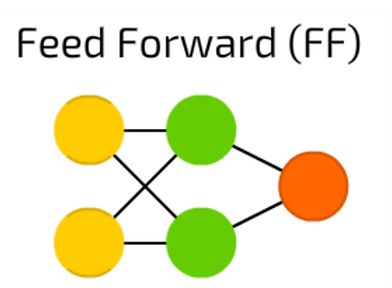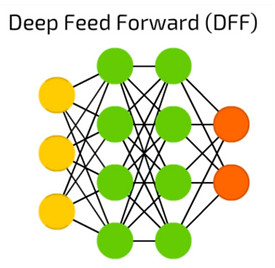top of page

## Neural Networks

What are neural networks

1. A set of algorithms or layers that recognize patterns and help to output similar outputs to logistic or linear regression

2. Neural networks help to find the relationship between the input and output

3. They can happen in both supervised learning and unsupervised learning. Some examples of neural networks can include facial recognition, voice identifiers, and spam emails

4. The unique part of neural networks is that it goes through numerous activation layers to get to the final layer

Deep Learning

1. A subset of machine learning that is typically utilized in unsupervised learning

2. More than one hidden layer

3. Each layer of nodes train on different features than the previous layer and the more the complex features the node recognizes and each layer takes the distinct features from the previous layer (known as feature hierarchy)

4. Deep learning can take numerous images and help put them together based on similar features. It can do the same thing with spam emails or spam calls.

Illustration of a neural network (Adapted from James Write from ResearchGate)Note that each type of neural network will have a different structure and that this is just an example

1. Each dot is a node and each node will have a structure such as the one shown below (Adapted from pathmind). Remember this same structure from logistic and kind of linear regression.## Below are vital concepts of Neural Networks

The Softmax Layer

1. Softmax is an activation function, part of the numerous layers, and is known as the outer layer.

2. Softmax layers limit the output to 0 to 1, allowing this output to be a probability. Notice that this is exactly what happens with a logistic regression algorithm. Therefore, using a softmax activation function means that you are using logistic regression. It is typically the final layer.

ReLu

1. ReLU, also known as rectified linear unit, is the most popular type of activation function and is used especially in Convolutional Neural Networks. Mathematically, it is known as:

• max(0,x)

Soft Plus

1. Activation = ln(ex+1)

2. This is the integral of the softmax layer and is useful in backpropogation (described below)

BackPropagation

1. Supervised learning using gradient descent. Back-propagation method calculates the best weights to use based on error in the previous epoch (an epoch is an iteration in a neural network)

2. Uses the derivative of the activation function

3. Called Back Propagation because the weights are updated backwards, from output to input

4. This is used mostly for feed forward neural networks (described later) in supervised learning

Hidden layer

1. Layer between input and output layers where they take a set of weighted inputs and produce an output through the activation function.

Optimization​

1. Optimization is essentially what type of function is computing the optimized weights. It typically uses back-propagation.

2. It needs a loss function to start with and tries to minimize it

3. An example optimization function that we will use in our application section frequently is rms prop which uses a continuously updated average of the squares of the previous gradients to compute a learning rate vector for each input instead of one for all inputs.

Regularization

1. This concept helps to avoid overfitting through stopping the model from becoming too complicated.

2. It further reduces the variance in the model without increasing the bias substantially.

HyperParameters

1.  Variables used to control the learning process. They are called HyperParameters because they have to be manually set and tuned         before the program is run.

2.  They govern the entire network structure.

3.  Some examples include the number of nodes in hidden layers or the optimization function.

What type of neural Networks are there and how do they look like:

1. This chart is popularly used to teach neural networks and their shapes. This sheet was taken from Fjodor Van Veen from the Asimov Institute:Now there will be examples of typical neural network structures as well as actual programming and separate pages for the two most popular ones.

Perceptron

1. Perceptron has no hidden layer, it just takes an input, dot products this with the weight, and goes to the output layer

2. The most simple neuron (The images of these networks have been adapted from Andrew Tch in Towards Data Science)Feed Forward Neural Network

1. All nodes are connected to eachother
2. There is only one hidden layer

3. There is no cycle and the network simply feeds forward with its inputs and weightsDeep Feed Forward Neural Network

1. Like a feedforward neural network but multiple hidden layers
2. This is the most popular type of neural network.

3. The foundation of Deep Learning networksRecurrent Neural Network

1. Used in language processing and speech recognition
2. Typically utilizes sigmoid and ReLU as activation functions

3. Measures the distance between a fixed point and an input and any point within that is in the Radial Basis, and is sliced and classified based on thatConvolutional Neural Network

Training and Validation Accuracy

1. Training Accuracy -  when you apply the model and backpropagation on training data

2. Validation Accuracy - applying the trained set of weights and test inputs on the test data

3. When the model starts to stagnate it overfits, the model is now memorizing the data and closely fit to a limited set of data points

4. A sample graph is shown below:bottom of page